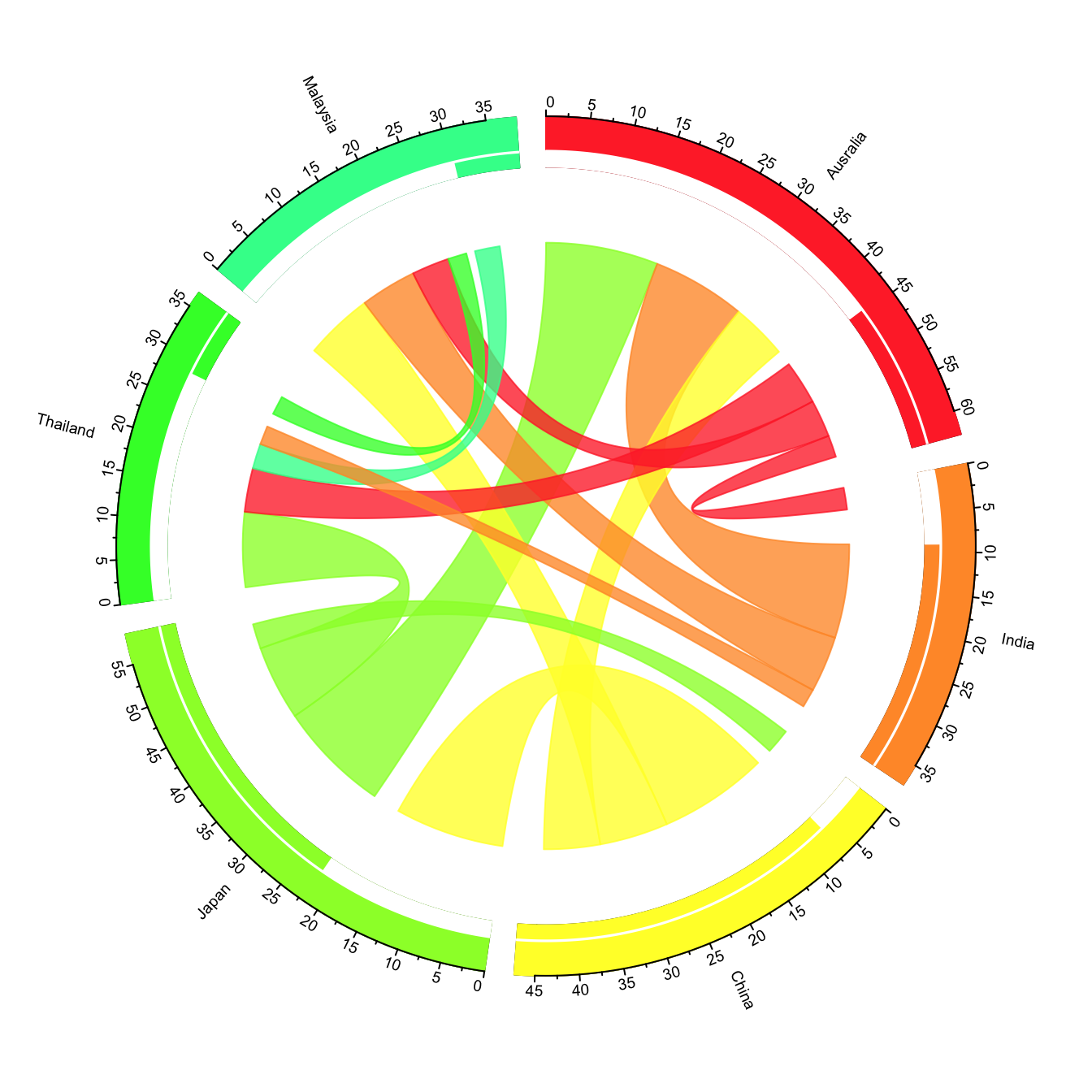# Advanced chord diagram with R and circlize

Chord diagram is an efficient way to display flows between entities. This post shows how to build a customized version using the circlize package.

# Chord diagram from adjacency matrix

The `circlize package` developped by Zuguang Gu is the best way to build chord diagram in R. The chord diagram section of the gallery provides a step by step introduction to it.

This example explains how to build a highly customized chord diagram, adding links manually thanks to the `circos.link()` function.

Note that the library also offers a `chordDiagram()` functions that builds everything automatically, but offers less customization. (See it here.)

Important: This example has been found on stackoverflow, made by Jazzuro.``````### You need several libraries
library(circlize)
library(migest)
library(dplyr)

### Make data
m <- data.frame(order = 1:6,
country = c("Ausralia", "India", "China", "Japan", "Thailand", "Malaysia"),
V3 = c(1, 150000, 90000, 180000, 15000, 10000),
V4 = c(35000, 1, 10000, 12000, 25000, 8000),
V5 = c(10000, 7000, 1, 40000, 5000, 4000),
V6 = c(7000, 8000, 175000, 1, 11000, 18000),
V7 = c(70000, 30000, 22000, 120000, 1, 40000),
V8 = c(60000, 90000, 110000, 14000, 30000, 1),
r = c(255,255,255,153,51,51),
g = c(51, 153, 255, 255, 255, 255),
b = c(51, 51, 51, 51, 51, 153),
stringsAsFactors = FALSE)
df1 <- m[, c(1,2, 9:11)]
m <- m[,-(1:2)]/1e04
m <- as.matrix(m[,c(1:6)])
dimnames(m) <- list(orig = df1\$country, dest = df1\$country)
#Sort order of data.frame and matrix for plotting in circos
df1 <- arrange(df1, order)
df1\$country <- factor(df1\$country, levels = df1\$country)
m <- m[levels(df1\$country),levels(df1\$country)]

### Define ranges of circos sectors and their colors (both of the sectors and the links)
df1\$xmin <- 0
df1\$xmax <- rowSums(m) + colSums(m)
n <- nrow(df1)
df1\$rcol<-rgb(df1\$r, df1\$g, df1\$b, max = 255)
df1\$lcol<-rgb(df1\$r, df1\$g, df1\$b, alpha=200, max = 255)

### Plot sectors (outer part)
par(mar=rep(0,4))
circos.clear()

### Basic circos graphic parameters
circos.par(cell.padding=c(0,0,0,0), track.margin=c(0,0.15), start.degree = 90, gap.degree =4)

### Sector details
circos.initialize(factors = df1\$country, xlim = cbind(df1\$xmin, df1\$xmax))

### Plot sectors
circos.trackPlotRegion(ylim = c(0, 1), factors = df1\$country, track.height=0.1,
#panel.fun for each sector
panel.fun = function(x, y) {
#select details of current sector
name = get.cell.meta.data("sector.index")
i = get.cell.meta.data("sector.numeric.index")
xlim = get.cell.meta.data("xlim")
ylim = get.cell.meta.data("ylim")

#text direction (dd) and adjusmtents (aa)
theta = circlize(mean(xlim), 1.3)[1, 1] %% 360
dd <- ifelse(theta < 90 || theta > 270, "clockwise", "reverse.clockwise")
aa = c(1, 0.5)
if(theta < 90 || theta > 270)  aa = c(0, 0.5)

#plot country labels
circos.text(x=mean(xlim), y=1.7, labels=name, facing = dd, cex=0.6,  adj = aa)

#plot main sector
circos.rect(xleft=xlim, ybottom=ylim, xright=xlim, ytop=ylim,
col = df1\$rcol[i], border=df1\$rcol[i])

#blank in part of main sector
circos.rect(xleft=xlim, ybottom=ylim, xright=xlim-rowSums(m)[i], ytop=ylim+0.3,
col = "white", border = "white")

#white line all the way around
circos.rect(xleft=xlim, ybottom=0.3, xright=xlim, ytop=0.32, col = "white", border = "white")

#plot axis
circos.axis(labels.cex=0.6, direction = "outside", major.at=seq(from=0,to=floor(df1\$xmax)[i],by=5),
minor.ticks=1, labels.away.percentage = 0.15)
})

### Add sum values to df1, marking the x-position of the first links
### out (sum1) and in (sum2). Updated for further links in loop below.
df1\$sum1 <- colSums(m)
df1\$sum2 <- numeric(n)

### Create a data.frame of the flow matrix sorted by flow size, to allow largest flow plotted first
df2 <- cbind(as.data.frame(m),orig=rownames(m),  stringsAsFactors=FALSE)
df2 <- reshape(df2, idvar="orig", varying=list(1:n), direction="long",
timevar="dest", time=rownames(m),  v.names = "m")
df2 <- arrange(df2,desc(m))

### Keep only the largest flows to avoid clutter
df2 <- subset(df2, m > quantile(m,0.6))

for(k in 1:nrow(df2)){
#i,j reference of flow matrix
i<-match(df2\$orig[k],df1\$country)
j<-match(df2\$dest[k],df1\$country)

circos.link(sector.index1=df1\$country[i], point1=c(df1\$sum1[i], df1\$sum1[i] + abs(m[i, j])),
sector.index2=df1\$country[j], point2=c(df1\$sum2[j], df1\$sum2[j] + abs(m[i, j])),
col = df1\$lcol[i])

#update sum1 and sum2 for use when plotting the next link
df1\$sum1[i] = df1\$sum1[i] + abs(m[i, j])
df1\$sum2[j] = df1\$sum2[j] + abs(m[i, j])
}``````

Related chart types

## Contact

This document is a work by Yan Holtz. Any feedback is highly encouraged. You can fill an issue on Github, drop me a message on Twitter, or send an email pasting yan.holtz.data with gmail.com.